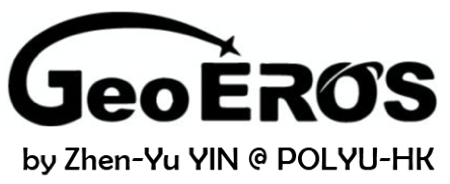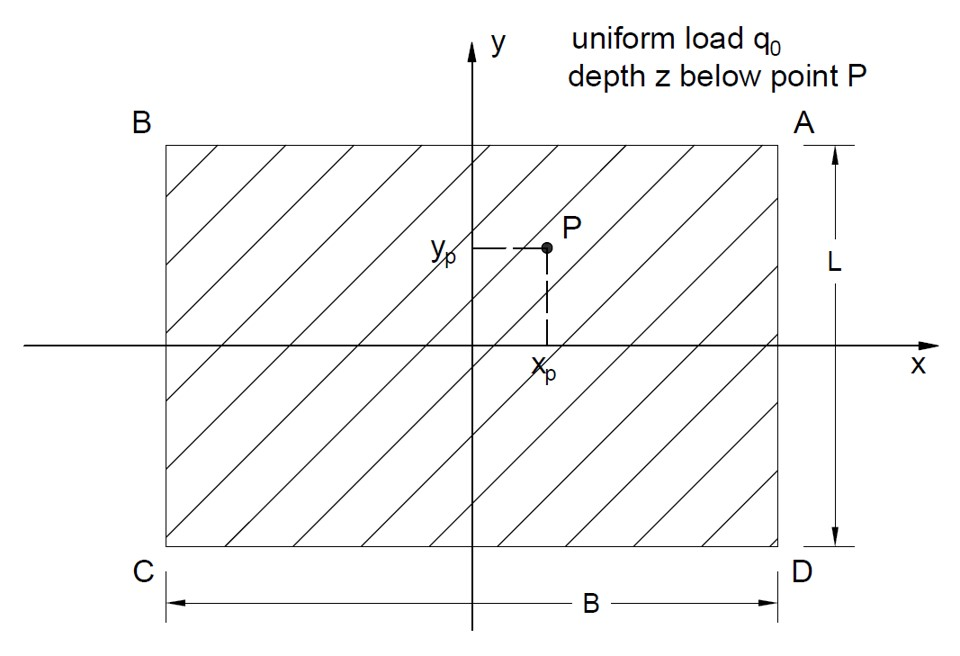# Rectangular Load Soil Stress Tool## Geometry of Uniform Load (plan view)## Tips

• This tool is for calculating vertical stress increment in soil induced by the applied uniform load, as shown in the figure above.
• The soil layer is assumed as homogeneous, elastic and isotropic.
• For the Boussinesq method, the vertical stress increment calculated is for the position at the depth z below the point P (xp,yp).
• For the approximate 2:1 method, the vertical stress increment calculated is only for the position at the depth z below the center point (0,0).
• All the input parameters except the coordinates of point P should be positive.
• For "nan", "0" or "inf" displayed in Results, please check your input parameters.

B (m)
L (m)
xp (m)
yp (m)
z (m)
q0 (kN/m2)